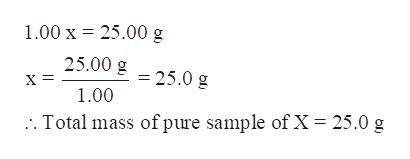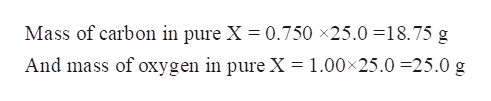# A pure sample of compound X contains only carbon and oxygen in a mass ratio of 0.750 g C : 1.00 g O. An impure sample of compound X has a total mass of 75.00 g and is found to contain 25.00 g oxygen. Assuming that the contaminants do not contain oxygen, determine the mass of impurities within the impure sample of Compound X.

Question
24 views

A pure sample of compound X contains only carbon and oxygen in a mass ratio of 0.750 g C : 1.00 g O. An impure sample of compound X has a total mass of 75.00 g and is found to contain 25.00 g oxygen. Assuming that the contaminants do not contain oxygen, determine the mass of impurities within the impure sample of Compound X.

check_circle

Step 1

A pure sample of compound X contains only carbon and oxygen in a mass ratio of 0.750 g C: 1.00 g O. An impure sample of compound X has a total mass of 75.00 g and is found to contain 25.00 g oxygen. Assuming that the contaminants do not contain oxygen, the mass of impurities within the impure sample of Compound X is to be determined.

Step 2

Let the total mass of the pure compound X be x.

Thus, mass of carbon in pure X = 0.750 x

And mass of oxygen in pure X = 1.00 x

Now, the 75.0 g of impure X has 25.00 g of oxygen and it is mentioned that impurities do not contain impurities.

Therefore, 1.00 x = 25.00

Solve for x.help_outlineImage Transcriptionclose1.00 x 25.00 g 25.00 g X = = 25.0 g 1.00 Total mass of pure sample of X 25.0 g fullscreen
Step 3

Calculate the mass of carbon and oxygen in pure sample-

Mass of carbon in pure X ...help_outlineImage TranscriptioncloseMass of carbon in pure X = 0.750 x25.0 18.75 g And mass of oxygen in pure X = 1.00x25.0 25.0 g fullscreen

### Want to see the full answer?

See Solution

#### Want to see this answer and more?

Solutions are written by subject experts who are available 24/7. Questions are typically answered within 1 hour.*

See Solution
*Response times may vary by subject and question.
Tagged in

### General Chemistry